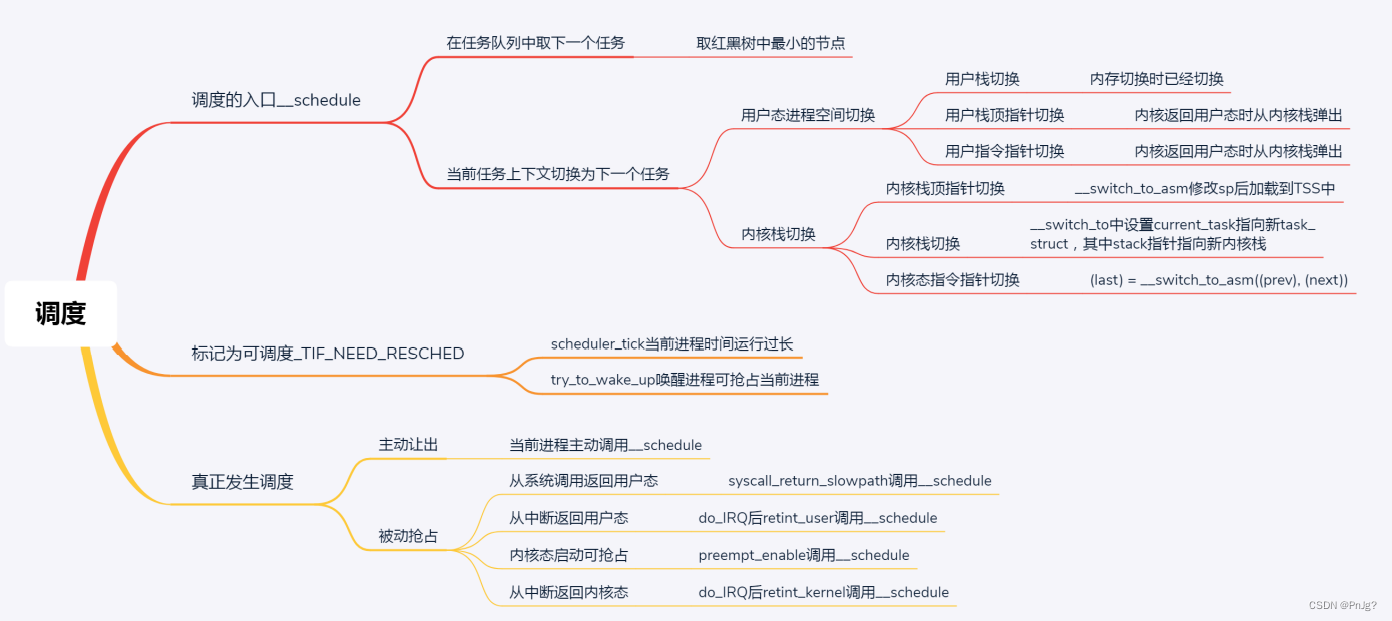# 抢占式场景一：

``````void scheduler_tick(void)
{
int cpu = smp_processor_id();
struct rq *rq = cpu_rq(cpu);
......
......
}``````

``````static void task_tick_fair(struct rq *rq, struct task_struct *curr, int queued)
{
struct cfs_rq *cfs_rq;
struct sched_entity *se = &curr->se;//当前运行对应的对象实体
for_each_sched_entity(se) {
cfs_rq = cfs_rq_of(se);//队列
entity_tick(cfs_rq, se, queued);
}
......
}``````

entity_tick的实现如下：

``````static void
entity_tick(struct cfs_rq *cfs_rq, struct sched_entity *curr, int queued)
{
update_curr(cfs_rq);
update_cfs_shares(curr);
.....
if (cfs_rq->nr_running > 1)
check_preempt_tick(cfs_rq, curr);
}
``````

check_preempt_tick实现如下：

``````static void
check_preempt_tick(struct cfs_rq *cfs_rq, struct sched_entity *curr)
{
unsigned long ideal_runtime, delta_exec;
struct sched_entity *se;
s64 delta;
ideal_runtime = sched_slice(cfs_rq, curr);
delta_exec = curr->sum_exec_runtime - curr->prev_sum_exec_runtime;
if (delta_exec > ideal_runtime) {
resched_curr(rq_of(cfs_rq));
return;
}
......
se = __pick_first_entity(cfs_rq);
delta = curr->vruntime - se->vruntime;
if (delta < 0)
return;
if (delta > ideal_runtime)
resched_curr(rq_of(cfs_rq));
}
``````

check_preempt_tick先是调用sched_slice函数计算出的ideal_runtime，他是一个调度周期中，这个进程应该运行的实际时间

sum_exec_runtime指进程总共执行的实际时间prev_sum_exec_runtime上次该进程被调度时已经占用的实际时间。每次在调度一个新的进程时都会把它的se->prev_sum_exec_runtime = se->sum_exec_runtime，所以sum_exec_runtime-prev_sum_exec_runtime就是这次调度占用实际时间。如果这个时间大于ideal_runtime，则应该被抢占了。

set_tsk_need_resched实现如下：

``````static inline void set_tsk_need_resched(struct task_struct *tsk)
{
}``````

# 抢占式场景二

``````static void ttwu_do_wakeup(struct rq *rq, struct task_struct *p, int wake_flags,
struct rq_flags *rf)
{
check_preempt_curr(rq, p, wake_flags);
trace_sched_wakeup(p);``````

# 抢占的时机

## 用户态的抢占时机

### 抢占式机一：

64位的系统调用的链路位do_syscall_64->syscall_return_slowpath- >prepare_exit_to_usermode->exit_to_usermode_loop。其中exit_to_usermode_loop这个函数实现如下：

``````static void exit_to_usermode_loop(struct pt_regs *regs, u32 cached_flags)
{
while (true) {
/* We have work to do. */
local_irq_enable();
if (cached_flags & _TIF_NEED_RESCHED)
schedule();
......
}
}
``````

exit_to_usermode_loop函数中，上面打的标记起了作用，如果被打了 _TIF_NEED_RESCHED，调用schedule进行调度，调用的过程和上一节解析的一样，会选择一个进程让出CPU，做上下文切换。

### 抢占时机二：

返回用户态这一部分retint_user会调用 prepare_exit_to_usermode，最终调用exit_to_usermode_loop，和上面的逻辑一样，发现有 标记则调用schedule()。

## 内核态的抢占时机

### 时机一

``````#define preempt_enable() \
do { \
if (unlikely(preempt_count_dec_and_test())) \
__preempt_schedule(); \
} while (0)

#define preempt_count_dec_and_test() \
({ preempt_count_sub(1); should_resched(0); })
static __always_inline bool should_resched(int preempt_offset)
{
return unlikely(preempt_count() == preempt_offset &&
tif_need_resched());
}
static void __sched notrace preempt_schedule_common(void)
{
do {
......
__schedule(true);
......
} while (need_resched())
``````

### 时机二

``````asmlinkage __visible void __sched preempt_schedule_irq(void)
{
......
do {
preempt_disable();
local_irq_enable();
__schedule(true);
local_irq_disable();
sched_preempt_enable_no_resched();
} while (need_resched());
......
}``````

preempt_schedule_irq调用__schedule进行调度。还是满足进程调度第一定律的。

# 总结